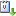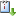Usted está aquí: A proof of a conjecture on bipartite Ramsey numbers B(2, 2, 3)

# A proof of a conjecture on bipartite Ramsey numbers B(2, 2, 3)

Ponente: Mostafa Gholami​
Institución: Institute for Advanced Studies in Basic Sciences, Zanjan, Iran
Tipo de Evento: Investigación
Cuándo 25/03/2022 de 12:00 a 13:00 meet.google.com/gnm-huaz-mwavCaliCal
The bipartite Ramsey number B(n_1,n_2,...,n_t) is the least positive integer b, such that any coloring of the edges of K_{b,b} with t colors will result in a monochromatic copy of K_{n_i,n_i} in the i-th color, for some i, 1 ≤ I ≤ t. The values B(2,5)=17,  B(2,2,2,2)=19 and B(2,2,2)=11 have been computed in several papers up to now.  We obtain the exact value of bipartite Ramsey number B(2,2,3). In particular, we prove  the conjecture on B(2,2,3) which was proposed in 2015. In fact we prove that  B(2,2,3)=17.